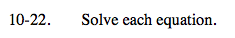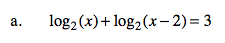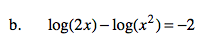### Home > A2C > Chapter Ch10 > Lesson 10.1.1 > Problem10-22

10-22.Remember the properties of logarithms.

log2(x(x − 2)) = 3
x 2 − 2x = 8
x 2 − 2x − 8 = 0
(x − 4)(x + 2) = 0

4, since logarithms of negative numbers are undefined.See part (a).